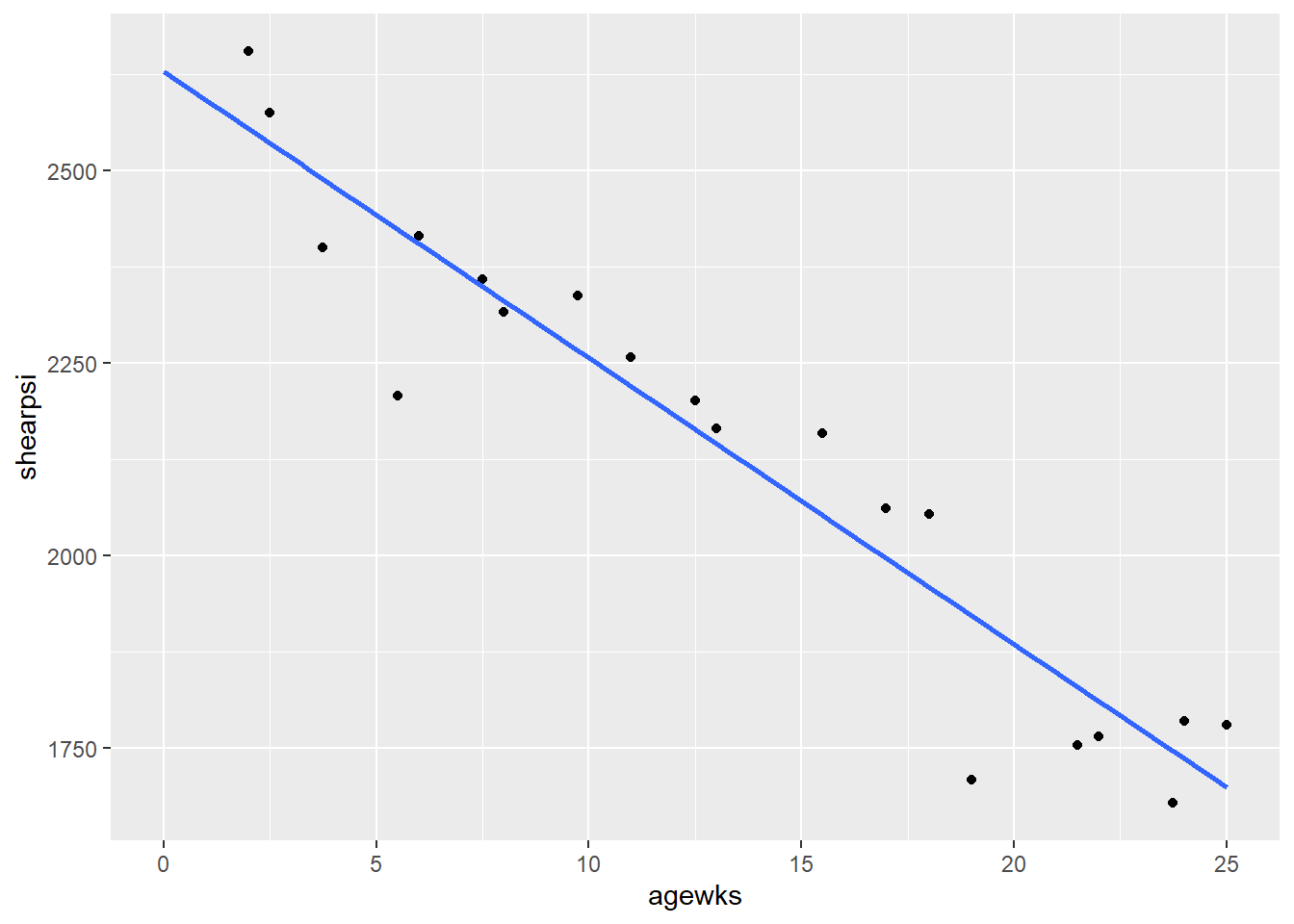# Rubric

Answer the questions in this document, compile to html, print to pdf, and submit to UNM Learn. Do not add this to your “ALL” .Rmd document.

## Part 1, simple linear regression intuition-building exercise

(20-25 min)

Use this online app to play with the next four challenges.

Complete each of steps and have a tablemate check your work for each. Give each other a thumbs up when you’ve got it!

1. Choose 3 points where the line of best fit is $$y = 0 + 1 x$$.
1. Click “Add Points” and click 3 times in the plot area.
2. Click “Display line of best fit” to show the red “best fit” line.
3. Click “Move Points” then move the points in the plot area.
4. Try to get within $$\pm 0.05$$ of the target intercept of 0 and slope of 1. Note that the app displays the equations slope before the intercept, as in $$y = 1 x + 0$$.
• When done, deselect “Display line of best fit” and click “Reset”.
2. Fit your own line to 7 points.
1. Click “Add Points” and click 7 times in the plot area.
2. Click “Fit your own line” to show the green “your own” line.
3. Click “Move Your Fit Line” then use the green circles on the line to move it.
4. Recall that the best line passes through the mean (center) of the data and minimizes the sum of squared error (in the $$y$$ direction).
5. Click “Display line of best fit” to see how close your line was to the red “best fit” line.
6. Repeat a couple times.
• When done, deselect “Fit your own line” and “Display line of best fit” and click “Reset”.
3. Illustrate the concept of leverage.
• “Leverage” is a measure of how much a point is an outlier (extreme) in the $$x$$ direction. It’s called leverage because points with high leverage potentially have a lot of influence on the regression line slope, pulling it up or down like a lever.
1. Click “Display line of best fit” to show the red “best fit” line.
2. Place 9 points in a “cluster” on one side of the plot.
3. Place 1 “solo” point by itself on the other side of the plot.
4. Move the “solo” point up and down and notice how the regression line responds.
5. Move one of the “cluster” points up and down and notice how the regression line responds.
6. Discuss this behavior with a tablemate.
• When done, deselect “Display line of best fit” and click “Reset”.
4. Relationship between correlation and slope.
1. Click “Add Points” and click 7 times in the plot area.
2. Click “Display line of best fit” to show the red “best fit” line.
3. Click “Move Points” then move the points in the plot area.
4. Make $$r < 0$$ and a best fit line with positive slope.

## Part 2, interpreting analysis

Refer to the data and output below these questions.

Answer the questions in this document, compile to html, print to pdf, and submit to UNM Learn.

1. (2 p) Write regression equation.

2. (2 p) Interpret slope.

3. (2 p) Interpret R2

4. (2 p) Complete this table of predictions.

Replace the question marks with values. You can use R as a calculator.

agewks shearpsi
5 ?
20 ?
40 ?
1. (2 p) Predictions: How comfortable do you feel (“good” or “bad”) about predictions for each of these values, and why?
• agewks = 5:
• agewks = 20:
• agewks = 40:

### Data and output

A rocket motor is manufactured by bonding an igniter propellant and a sustainer propellant together inside a metal housing. The shear strength of the bond between the two types of propellant is an important quality characteristic. It is suspected that shear strength is related to the age in weeks of the batch of sustainer propellant. Twenty observations on these two characteristics are given below. The first column is shear strength in psi (shearpsi), the second is age of propellant in weeks (agewks).

## Save the Rmd and .dat the ADA_WS_09_Data-BrainSizeData.csv data file to your computer

# this file uses spaces as delimiters, so use read.table()
str(rocket)
## 'data.frame':    20 obs. of  2 variables:
##  $shearpsi: num 2159 1678 2316 2061 2208 ... ##$ agewks  : num  15.5 23.8 8 17 5.5 ...
head(rocket)
##   shearpsi agewks
## 1  2158.70  15.50
## 2  1678.15  23.75
## 3  2316.00   8.00
## 4  2061.30  17.00
## 5  2207.50   5.50
## 6  1708.30  19.00
library(ggplot2)
p <- ggplot(rocket, aes(x = agewks, y = shearpsi))
p <- p + geom_point()
p <- p + geom_smooth(method = lm, se = FALSE, fullrange = TRUE)
p <- p + xlim(0,NA)
print(p)# fit the simple linear regression model
lm.shearpsi.agewks <- lm(shearpsi ~ agewks, data = rocket)
# use summary() to parameters estimates (slope, intercept) and other summaries
summary(lm.shearpsi.agewks)
##
## Call:
## lm(formula = shearpsi ~ agewks, data = rocket)
##
## Residuals:
##     Min      1Q  Median      3Q     Max
## -215.98  -50.68   28.74   66.61  106.76
##
## Coefficients:
##             Estimate Std. Error t value Pr(>|t|)
## (Intercept) 2627.822     44.184   59.48  < 2e-16 ***
## agewks       -37.154      2.889  -12.86 1.64e-10 ***
## ---
## Signif. codes:  0 '***' 0.001 '**' 0.01 '*' 0.05 '.' 0.1 ' ' 1
##
## Residual standard error: 96.11 on 18 degrees of freedom
## Multiple R-squared:  0.9018, Adjusted R-squared:  0.8964
## F-statistic: 165.4 on 1 and 18 DF,  p-value: 1.643e-10